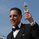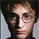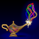4539 obejrzeń
This indicator plots the moving average described in the January, 1998 issue
of S&C, p.57, "Smoothing Techniques for More Accurate Signals", by Tim Tillson.
This indicator plots T3 moving average presented in Figure 4 in the article.
T3 indicator is a moving average which is calculated according to formula:
T3(n) = GD ( GD ( GD (n))),
where GD - generalized DEMA ( Double EMA ) and calculating according to this:
GD (n,v) = EMA (n) * (1+v)-EMA( EMA (n)) * v,
where "v" is volume factor, which determines how hot the moving average’s response
to linear trends will be. The author advises to use v=0.7.
When v = 0, GD = EMA , and when v = 1, GD = DEMA . In between, GD is a less aggressive
version of DEMA . By using a value for v less than1, trader cure the multiple DEMA
overshoot problem but at the cost of accepting some additional phase delay.
In filter theory terminology, T3 is a six-pole nonlinear Kalman filter. Kalman
filters are ones that use the error — in this case, (time series - EMA (n)) —
to correct themselves. In the realm of technical analysis , these are called adaptive
moving averages; they track the time series more aggres-sively when it is making large
moves. Tim Tillson is a software project manager at Hewlett-Packard, with degrees in
mathematics and computer science. He has privately traded options and equities for 15 years.
```////////////////////////////////////////////////////////////
//  Copyright by HPotter v1.0 21/05/2014
// This indicator plots the moving average described in the January, 1998 issue
// of S&C, p.57, "Smoothing Techniques for More Accurate Signals", by Tim Tillson.
// This indicator plots T3 moving average presented in Figure 4 in the article.
// T3 indicator is a moving average which is calculated according to formula:
//     T3(n) = GD(GD(GD(n))),
// where GD - generalized DEMA (Double EMA) and calculating according to this:
//     GD(n,v) = EMA(n) * (1+v)-EMA(EMA(n)) * v,
// where "v" is volume factor, which determines how hot the moving average’s response
// to linear trends will be. The author advises to use v=0.7.
// When v = 0, GD = EMA, and when v = 1, GD = DEMA. In between, GD is a less aggressive
// version of DEMA. By using a value for v less than1, trader cure the multiple DEMA
// overshoot problem but at the cost of accepting some additional phase delay.
// In filter theory terminology, T3 is a six-pole nonlinear Kalman filter. Kalman
// filters are ones that use the error — in this case, (time series - EMA(n)) —
// to correct themselves. In the realm of technical analysis, these are called adaptive
// moving averages; they track the time series more aggres-sively when it is making large
// moves. Tim Tillson is a software project manager at Hewlett-Packard, with degrees in
// mathematics and computer science. He has privately traded options and equities for 15 years.
////////////////////////////////////////////////////////////
study(title="T3 Averages", shorttitle="T3", overlay = true)
Length = input(5, minval=1)
xPrice = close
xe1 = ema(xPrice, Length)
xe2 = ema(xe1, Length)
xe3 = ema(xe2, Length)
xe4 = ema(xe3, Length)
xe5 = ema(xe4, Length)
xe6 = ema(xe5, Length)
b = 0.7
c1 = -b*b*b
c2 = 3*b*b+3*b*b*b
c3 = -6*b*b-3*b-3*b*b*b
c4 = 1+3*b+b*b*b+3*b*b
nT3Average = c1 * xe6 + c2 * xe5 + c3 * xe4 + c4 * xe3
plot(nT3Average, color=blue, title="T3")
```

## KomentarzeHi Harry! Great Work!!!

I found this one looking for a DEMA with a setting so that it can be applied to high-low-open-close prices. Seems there's none in Tradingview.... Do you think you could help me?

OdpowiedzDiegoKatzman
Hi Diego,
If I understand you correctly, you can change a line "xPrice = close" on the "xPrice = ohlc4"
OdpowiedzHPotter
Thanks! I meant getting just the adjustable DEMA in a separate indicator :D

Do you think you could create one from your script?
OdpowiedzHi HP: I just read an article in S&C 2010 where a Kalman filter was utilized to predict or forecast future price trends. Could this indicator be extended to accomplish the same goal?
You can find the article here: http://edmond.mires.co/GES816/22-Predicting%20Market%20Data%20Using%20The%20Kalman%20Filter(1).pdf
OdpowiedzStockSwinger
How do you plan on using the Kalman? Ive tried using it for quite some time in different manners. In theory it sounds great. But in my experience, I found it difficult to implement in a reliable and efficient way.
Odpowiedzcooney_s
HI CD71:

From what I took away from the article the formula can be utilized to project forward what the next several day price actions will be. If you can do that with even a 60% chance of being correct it would be a dramatic improvement of a 50/50 coin flip. HP was utilizing the Kalman code to smooth out a moving Avg, not to forecast which is my intent. I fully realize that forecasting is like predicting the weather with magic, but I got the time to invest to see if there might be some magic in this formula. Ehlers Center of Gravity come pretty close.
OdpowiedzThis is a great indicator. Can we please request an Oscillator version. I would like to overlap several T3 averages with different volume factors. Much cleaner to read the momentum within an oscillator. Thanks! Great work.
Odpowiedzcooney_s
"Can we please request an Oscillator version." Do you have a formula? I can try make it.
Odpowiedz